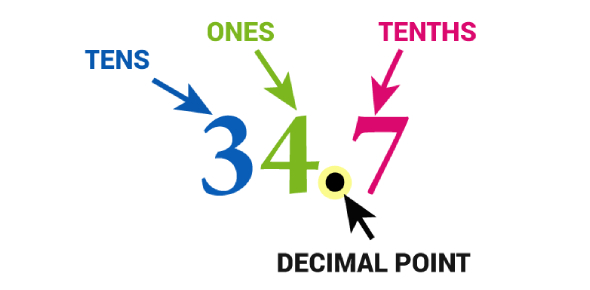# Decimals Quiz For Grade 5

10 Questions | Total Attempts: 15837SettingsDo you understand the importance of learning decimals? This Decimals quiz for grade 5 will show you how to solve problems that contain numbers with decimals. Decimals are valuable because we use them in everyday situations, such as dealing with money, reading the odometer, reviewing Olympic scores, or teaching your children how to add or subtract them. The decimal point emphasizes large and small numbers by employing the decimal point. Do you get the point? Take the quiz.

• 1.
0.163 + 146 =
• A.

146

• B.

146.163

• C.

163.146

• D.

147.63

• 2.
85.8 + 971 =
• A.

1067.1

• B.

1065

• C.

1058

• D.

1056.8

• 3.
33.6 + 98.3 =
• A.

131.9

• B.

132.9

• C.

132.98

• D.

133

• 4.
99.98 - 6.88 =
• A.

93.1

• B.

93.01

• C.

93.88

• D.

None of these

• 5.
86.366 - 86.3 =
• A.

0

• B.

66

• C.

0.066

• D.

None of these

• 6.
15.35 - 9.74 =
• A.

56.1

• B.

5.61

• C.

561

• D.

None of these

• 7.
0.278 × 0.15 =
• A.

0.781

• B.

782

• C.

31

• D.

None of these

• 8.
6.3 × 0.18 =
• A.

11.34

• B.

113.4

• C.

1.134

• D.

1134

• 9.
1.4 × 15 =
• A.

21

• B.

22

• C.

22.5

• D.

24

• 10.
226.24 ÷ 56 =
• A.

40.4

• B.

4.04

• C.

0.404

• D.

None of these

Related TopicsBack to top